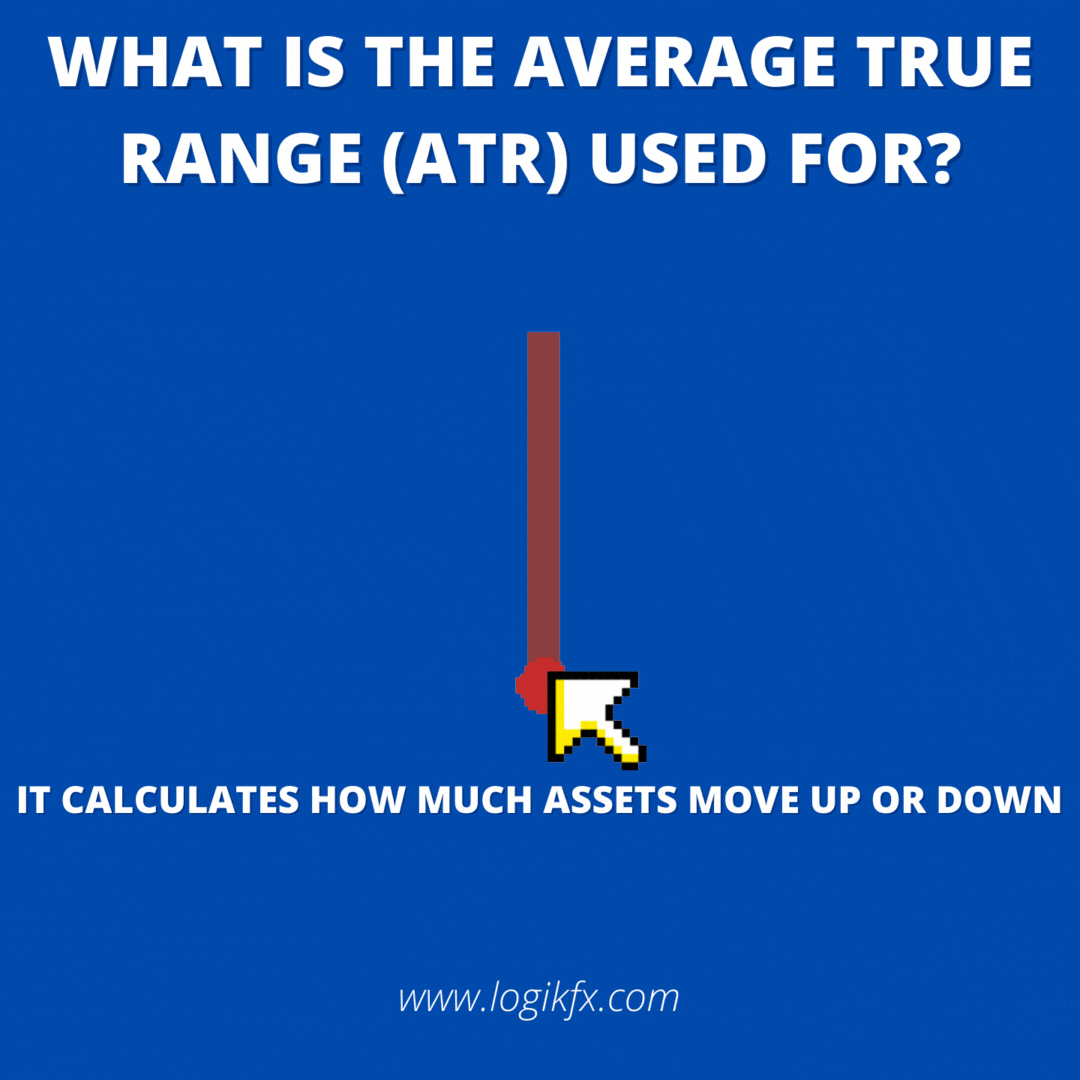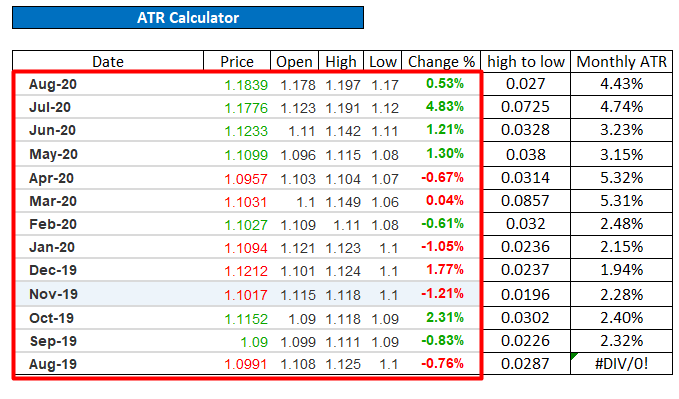top of page

# ATR Stop Loss Calculator- 5 Step Guide to Trading using Average True Range

Updated: Nov 1, 2022## How to read and use the Average True Range (ATR)

The simplest way to describe the Average True Range ATR calculation is to measure market volatility. Don’t worry if you don’t know what this means right now in a newbie term all it’s doing is measuring how much an asset goes up and down using maths!

It's important because as professional traders we don't want to be setting static stop losses or take profits based on pips! This is the wrong approach and you'll likely get stopped out a lot because you don't actually know how much the currency pair moves.

Using an ATR calculation solves this by professional setting stops based on volatility of currency pairs. There will be a unique ATR for each thing you trade so make sure to read this article fully to understand why you're getting stopped out.

Common misconceptions about the Average True Range ATR and ATR Indicators is that there’s some sort of strategy behind it. There’s not. All it’s doing is measuring the volatility and not telling us which direction the price is likely to move.What is average true range ATR

What you’ll actually be able to do after reading this short article is work out yourself how much prices move over given time frames. This is much better than relying on some shoddy indicator you find on platforms, at least this way you’re in full control!

You might realise after reading this article if an ATR is 3% within a month and your stops are less than this, very likely you'll be getting stopped out so start using ATR in your trading NOW! It's so important..

## Key Points for using the Average True Range (ATR)

• How to use ATR for stop losses and take profits

• How to use volatility to improve profitability

• Moving away from placing stop losses based on pips and candles but on volatility (ATR)

• Understand why setting stop losses and take profits with pips is bad

## How To Calculate Average True Range (ATR)

Below is the formula for how to calculate the average true range, but for the people that don't like maths like me I've made an easy to use ATR stop loss calculator.

TR = Max[(HL), Abs(HCP​), Abs(LCP​)]
ATR = (n1​)(i=1)∑(n)​TRi
Where:
TRi = A particular true range
n = The time period employed​

Overall, the formula uses a series of true range values of an asset or security. The range of an asset for a trading day is the high minus its low. Now that you're bored of the maths, let's take a look at the easy method to calculate the average true range ATR calculator to set stop losses and take profit targets.

Enough of the boring maths formula for ATR!

Calculating the Average True Range (ATR) is really easy because we’ve made a simple ATR calculator template for you so literally all you need to do is copy and paste price data into the cells and it does all the grunt work for you. It’s currently on sale so don’t miss out use coupon code “ATR99” to get up to 99% off.

If the ATR Stop Loss Calculator excel template is what you want to use then go ahead and click the image below to download the spreadsheet.

You want to learn how to create an ATR stop loss calculator for free, then continue reading the article!

## How to Create the Average True Range (ATR) Stop Loss Calculator

This is what the ATR Stop Loss Calculator looks like:What we’ve got in the calculator is price data, the average monthly volatility and then a calculator to automatically workout our stop losses for long positions and short positions!

So it’s now really easy to work out the stop loss (SL) and take profit (TP) every week.

Confusing? Let’s break it down so you understand how it works and how you can do it too. On the left hand side we’ve got 12 months of price data to work out our volatility. This includes the Date, price, open, high, low and the % change.

## Step 1: Copy and paste price data for the "Date, Price, Open, High, Low and Change %"Above is the monthly data that I've gathered from investing.com historical price data for an asset. I've captured the past 13 data points so October 2020-September 2019.

If you wanted to work out the ATR and volatility of lower time frames you'd collect the data for the above for that time frame.

The next step is to calculate the high to low.

## Step 2: Calculate the "High to low"

To calculate the high to low we use the price data which we copy and pasted from google. This is the range of the month using this calculation:

(High - Low) = high to low
E.g. (19.03 - 18.6) = 0.3859Now that we have the high to low prices we’ve got the range of that month and we want to work out the average monthly volatility Average True Range (ATR). So we need to also do the same thing for all the months in the periods you've collected.

## Step 3: Calculate the Monthly Volatility with Average True Range (ATR)

Now we have all the components to create a monthly ATR to see the volatility of that month. This can be seen in Column "I" next to column "H".The calculation for the monthly ATR is as follows:

(Current high to low - previous high to low)/2/ Previous Price

On the excel example above, this would be the following excel formula:

=(SUM(H5:H6)/2/D6)

Now that we have calculated that monthly ATR we can drag the formula down for the rest of the months to autofill the spreadsheet.

The next step is to calculate the overall average volatility over 6 months and a year. This gives us a good indicator of how much we can expect the asset to move over each month in the course of a year or 6 months.

## Step 4: Calculate the Monthly Average True Range (6 - 12 month average)

Now that we’ve got the monthly ATR values for the past 12 months of price data we can work out an average over the past 6 to 12 months to use in our stop loss and take profit calculations.This is done really easily by just averaging the monthly ATR value of the past 6 months and 12 months which gives us around 4%.

You can use this formula:

=AVERAGE(Month 1 to 6) or =AVERAGE(Month 1 to 12)
E.g. =AVERAGE(I1:I10) on excel

So now we know over around 6 to 12 months the price will move around 4% on a monthly basis.

## Step 5: Calculate your Stop Loss and Take Profit Targets

The next step in the ATR calculator online is to just manually type in the current price of whatever you’re trading. For example if the EURUSD exchange rate was 18.75 I type 18.75 in the Exchange rate cell as pointed out above. This will answer the question on how to use ATR to set stop loss.The ATR stop loss for a long position is calculated by using the currency exchange rate and multiplying it by 1 minus the Average 1 month ATR over the year (the 4% mentioned earlier). We minus it from 1 because we want to work out a number that’s less than the current exchange rate.

Long Stop Loss = Exchange Rate * (1 - Average Monthly ATR)

Then the target is easy... it's just the current exchange rate multiplied by 1 plus 3 times the average monthly ATR over the year. This in the end works out as a 1:3 risk-reward stop loss and target based on volatility!

Long Take Profit Target = Exchange Rate * (1 + Average Monthly ATR)The short side of the calculator is worked out the same but inverted the operator for stop loss and take profit (soft target).

Short Stop Loss = Exchange Rate * (1 + Average Monthly ATR)
Short Take Profit Target = Exchange Rate * (1 - Average Monthly ATR)

On a graph this is what the final values would look like:Remember our excel calculator does this instantly so you don’t need to worry about doing the excel formulas. All you need to do is copy and paste price data into the price cells. If you don’t know how to do that I’ll show you now.

## How to update the ATR Stop Loss Calculator

### Step 1 - Find what asset you want the ATR for

In this case I’m just going to use EUR/USD exchange rate as an example. So, what you want to do is to type into google “investing.com EUR/USD historical data”. If you want to work it out for a different asset or currency pair just replace EUR/USD with whatever it is you want. It should look something like this.Go ahead and click the link. This will direct you to the website in which you can get free historic data. Our next step is to convert the data into monthly data. Head to the drop down menu on the left under the historic data title and click monthly. It will convert all data into monthly formats.### Step 2 - Copy and paste into the ATR Stop Loss calculator

Now we can go ahead and copy and past the past 12 months into our excel template. All we do is highlight current month data to this month last year like below. Right click and copy the data and then paste it in the excel template.

Here we've copied the last 12 months data.Then we've pasted it into the price section of the calculator.Now the data is in the red box area above our formulas automatically calculate everything else and we’ve got a stop loss and target to work with!

### Step 3 - Use the stop loss and take profit targets

Now that we’ve got new price data all we need to do is use the numbers from our calculator to plug into our trade ideas. Manually type in the exchange rate cell the current exchange rate of what you want to trade and you’ll automatically have the stop loss and take profit numbers you want to use in your trading!Remember to get your Average True Range ATR calculator template to work out stop losses and take profits professionally. Use coupon code “ATR99” for up to 99% off while the sale lasts.

Remember to comment below any thoughts and share with other traders!

Still learning how to trade? Learn through Logikfx Investment and Trading Academy (LITA) and take the first steps into growing your value as a trader with our free online courses, webinars, seminars. All from a small team of highly skilled traders with over 15 years’ experience in the financial markets. Learn how to make money trading forex, alongside the best ways to manage your risk through a proper trading journal, and sensible approaches to setting a stop loss (that doesn't get hit)!

Already know how to trade? Save hundreds of hours each month on trading technology, analysis and research using Logikfx's Macro Technology in the LITA Portal. Computing thousands of fundamental reports for over 23 economic regions, you'll know accurate currency strength at the click of a button.

15,519 views